20.2
20.1
19.2
19.1
18.2
18.1
17.2

# Diagram

In this section, the topic below describes the main concepts for using a diagram in the ASP.NET Chart Control.

And, the following documents detail specifics of each diagram type available.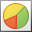Simple Diagram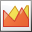XY-Diagram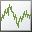Swift Plot Diagram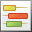Gantt Diagram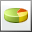Simple Diagram 3D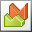XY-Diagram 3D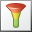Funnel Diagram 3D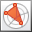Radar and Polar Diagrams